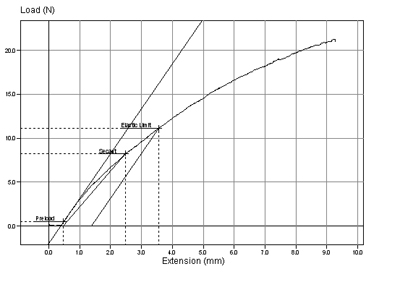## Elastic Limit Testing

Posted on July 12, 2016

Determining the elastic limit of a material involves the measurement of the greatest stress that can be applied to a sample without causing permanent deformation.

Metals or other rigid materials with a straight-line portion in their stress/strain curve have an elastic limit approximately equal to the proportional limit. Arbitrary approximation of the elastic limit of materials that do not have a significant straight-line portion on a stress/strain curve, such as rubber or plastic, is called Apparent Elastic Limit. It is equal to the stress at which the rate of strain is 50% greater than at zero stress. It is the stress at the point tangent between the stress-elastic hysteresis strain curve and the line having a slope, with respect to the stress axis, 50% greater than the slope of the curve at the origin.

Typical graph showing elastic limit test:Categories:Testing Types

Applications:Elastic Limit Testing